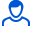Content: teorver_5583.doc (28.00 KB)

Positive responses: 0
Negative responses: 0

Refunds: 05583. x̅=116.68, n=36, σ=6. Find the confidence interval for estimating with a reliability of 0.99 the unknown mathematical expectation a of a normally distributed feature X of the general population, knowing the sample mean x̅=116.68, the sample size n=36 and the standard deviation σ=6.
Detailed solution. Decorated in Microsoft Word 2003 (Quest decided to use the formula editor)
No feedback yet